# JP4932641B2 - Peak power reduction device in communication device - Google Patents

## Info

Publication number
JP4932641B2
JP4932641B2 JP2007220751A JP2007220751A JP4932641B2 JP 4932641 B2 JP4932641 B2 JP 4932641B2 JP 2007220751 A JP2007220751 A JP 2007220751A JP 2007220751 A JP2007220751 A JP 2007220751A JP 4932641 B2 JP4932641 B2 JP 4932641B2
Authority
JP
Japan
Prior art keywords
phase rotation
phase
pattern
peak power
means
Prior art date
Legal status (The legal status is an assumption and is not a legal conclusion. Google has not performed a legal analysis and makes no representation as to the accuracy of the status listed.)
Expired - Fee Related
Application number
JP2007220751A
Other languages
Japanese (ja)
Other versions
JP2009055395A (en
Inventor

Original Assignee
ソフトバンクモバイル株式会社
Priority date (The priority date is an assumption and is not a legal conclusion. Google has not performed a legal analysis and makes no representation as to the accuracy of the date listed.)
Filing date
Publication date
Application filed by ソフトバンクモバイル株式会社 filed Critical ソフトバンクモバイル株式会社
Priority to JP2007220751A priority Critical patent/JP4932641B2/en
Publication of JP2009055395A publication Critical patent/JP2009055395A/en
Application granted granted Critical
Publication of JP4932641B2 publication Critical patent/JP4932641B2/en
Application status is Expired - Fee Related legal-status Critical
Anticipated expiration legal-status Critical

## Images

•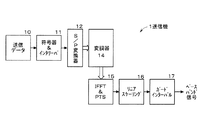•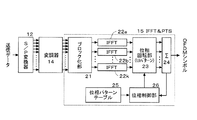•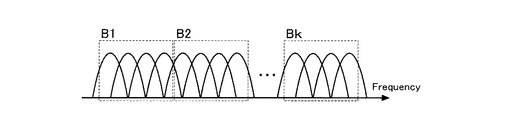•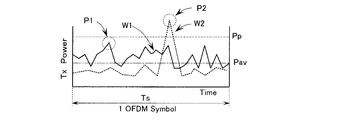•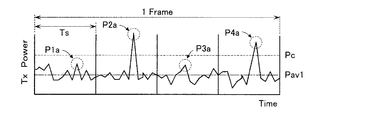•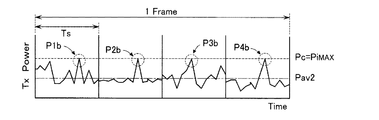•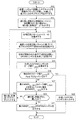•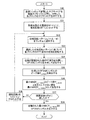•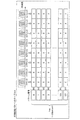•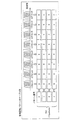•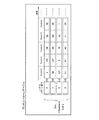•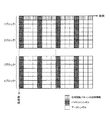•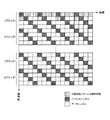•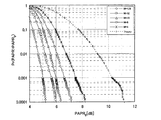•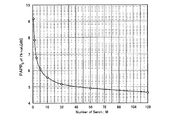•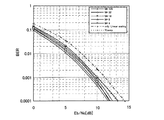•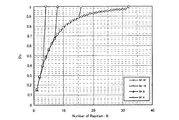•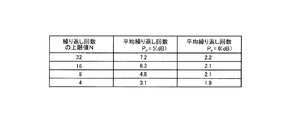•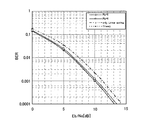## Description

The present invention relates to a peak power reduction device that reduces transmission peak power in a communication device that uses multicarriers.

Currently, MC-CDMA (multi-carrier code division multiple access) and OFDM (Orthogoal Frequency Division Multiplex) are known as communication systems using multicarriers. In the MC-CDMA system, since it is spread in the time domain, co-channel interference is reduced, and the OFDM system is used as a digital broadcast or wireless LAN system, and is regarded as a promising candidate for the next generation mobile communication system. Since this OFDM system is multi-carrier transmission, it has high frequency utilization efficiency and is resistant to frequency selective fading. In addition, the influence of multipath can be reduced by providing a guard interval (GI). By the way, in a communication system using multicarriers, modulation signals of a plurality of subcarriers are combined, so that the transmission peak power is higher than that of a single carrier modulation signal. In particular, since the peak power of an OFDM signal in which subcarriers orthogonal to each other are combined exhibits a larger peak power value than a conventional single carrier modulation signal, the peak power versus average power (PAPR) of a transmission signal is As a result, the transmission quality deteriorates. Furthermore, when the peak power increases, the power amplifier is required to increase the back-off that is the difference between the average value of the transmission signal power and the maximum transmission power of the power amplifier. It is necessary to use a power amplifier having a large maximum transmission power, resulting in a decrease in power efficiency, an increase in power consumption, and an increase in cost.

In order to solve this problem, various peak power reduction methods have been studied in the past. For example, a clipping method that distorts the signal in advance so that the peak power value becomes the set value, a coding method that uses an error correction code so that a code word with high peak power is not detected, and a phase rotation of the modulation signal of each subcarrier A selected mapping method (Selected Mapping) for optimal phase rotation from a plurality of phase rotation pattern candidates, and the inverse fast Fourier transform (IFFT) by dividing subcarriers into blocks in order to reduce the number of calculation processes of the selective mapping method ) A partial sequence transmission method (PTS: Partial Transmit Sequence) that rotates the phase later, and a linear scaling method that linearly compresses and amplifies the amplitude of the transmission signal.
The processing contents of partial sequence transmission (PTS) will be described. In PTS, first, subcarriers modulated with transmission symbols subjected to primary modulation are divided into B blocks. Next, the divided subcarriers are subjected to IFFT for each block to synthesize the subcarriers of each block. Phase rotation is performed for each block on the synthesized time axis waveform, the time axis waveforms subjected to phase rotation are added to generate an OFDM symbol, and the OFDM symbol waveform is stored in a memory.

Here, when the number of phase rotation candidates is L, L B (= U) patterns are obtained as the number of phase rotation patterns. Therefore, after performing phase rotation in all of the U patterns with respect to the time axis waveform after IFFT, an OFDM symbol waveform obtained by adding the time axis waveforms in each pattern is stored in the memory. The sample of the highest peak power value P i, uMAX (i: symbol number, u: pattern number (u = 1, 2... U)) is selected from the OFDM symbol waveforms of each pattern stored in the memory. P i, uMAX in each of the patterns is compared, and the minimum peak power value P iMAX (= MIN [P i, uMAX ]) is selected, and the OFDM symbol of the selected pattern is transmitted. In order to return the OFDM symbol to the waveform signal before the phase rotation on the receiver side, the phase rotation pattern is transmitted to the receiver side. On the receiver side, based on the control information of the phase rotation pattern sent from the transmission side, the waveform signal of the original phase is restored by performing phase rotation opposite to that on the transmission side.

Next, the linear scaling method will be described. If only linear compression is performed in the OFDM system, the reception power of the received symbol is reduced as it is, and the reception characteristics are deteriorated. However, transmission quality can be improved by performing linear compression and amplification until the peak value of the OFDM symbol reaches a desired threshold within the frame constituting the error correction code to improve the reception power. . When performing linear scaling, IFFT is applied to the transmission symbol to obtain a transmission waveform on the time axis. Next, the peak power P iMAX (i: symbol number) of each symbol is compared with a predetermined threshold value Pc from the obtained transmission waveform, and the case where the peak power P iMAX is larger than the threshold value Pc is applicable. By multiplying each symbol by a coefficient α i (<1) and reducing the transmission power, the transmission power is linearly compressed for each symbol so that the peak power P iMAX becomes the threshold value Pc. When the peak power P iMAX is lower than the threshold value Pc, transmission is performed by multiplying the coefficient α i (> 1) for each symbol so that the peak power P iMAX of each symbol becomes the threshold value Pc. The power is linearly amplified for each symbol. Here, α i = Pc / P iMAX . In this way, in linear scaling, each OFDM symbol is linearly compressed or amplified so that the peak power P iMAX of each transmission symbol is equal to the threshold value Pc, so that PAPR is reduced without being affected by out-of-band radiation. Can be achieved.

In the case of the conventional PTS method, the peak power reduction effect can be improved by setting a small step size of the phase coefficient or setting a large number of blocks in order to optimize the combination of phases. However, if the step size is set small or the number of blocks is set large, the number of candidates for phase rotation increases, and the number of multiplications increases exponentially. Therefore, when the PTS method is actually applied, an extremely large arithmetic circuit for performing multiplication of phase rotation candidates is required, particularly for mobile stations that are strongly required to reduce the size of the apparatus and reduce power consumption. It becomes difficult to apply.

In order to solve this problem, a peak power reduction method has been proposed that can reduce the number of multiplications of phase rotation candidates and can reduce the size of the arithmetic circuit and power consumption. In this peak power reduction method, a predetermined number of subcarrier modulation signals are multiplied by a plurality of candidate phase coefficients, respectively, when combining by sequentially adding a predetermined number of all subcarrier modulation signals. In addition to adding the subcarrier modulation signal to be added, the combination of the subcarrier modulation signals having the minimum peak power is selected from the addition result, and the combination of the selected subcarrier modulation signals is subjected to the next subcarrier modulation to be added. Signal processing is performed for all subcarrier modulation signals.
JP 2006-229746 A

However, in the conventionally proposed method for reducing peak power using the PTS method, in order to derive the optimum peak power, the number of blocks for dividing the subcarrier is increased or the phase rotation amount of the phase rotation amount is increased. When the number of patterns is increased, the number of adders that sequentially add a predetermined number of all subcarrier modulation signals and the number of selectors that select combinations of subcarrier modulation signals that minimize the peak power from the addition results are increased. It will increase exponentially. Therefore, there is a problem that a high-speed and high-performance processing device is required to cope with an increase in the amount of arithmetic processing. In the linear scaling method, linear compression or linear amplification is performed according to the peak power value. Therefore, if a transmission symbol having a high peak power value is linearly compressed to a desired threshold value, the compression rate becomes too large. Therefore, the average value of the transmission power of the transmission symbol is lowered and the transmission quality is deteriorated, so that there is a problem that the BER (Bit Error Rate) characteristic is deteriorated.

Accordingly, an object of the present invention is to provide a peak power reduction device in a communication device that does not require a high-speed and high-performance processing device and can reduce peak power without deteriorating transmission quality.

The peak power reduction device in the communication apparatus of the present invention capable of achieving the above object is a phase control for controlling the phase of the composite waveform corresponding to each block for each composite waveform so that the peak value in the composite waveform is reduced. And a linear scaling means for adjusting the gain of the symbol waveform for each OFDM symbol so that the peak value in the symbol waveform becomes a predetermined second threshold value. Selection means for sequentially selecting a phase rotation pattern from among a plurality of phase rotation patterns that are combinations obtained by assigning at least two candidates for phase rotation to each block, and according to the phase rotation pattern selected by the selection means Phase rotation means for rotating the phase of each composite waveform and the peak power value of the symbol waveform output from the addition means Detecting means for detecting, and when the detecting means detects that the peak power value of the symbol waveform is equal to or lower than a preset first threshold value, the selection of the phase rotation pattern is terminated and added at that time The most important feature is that the symbol waveform output from the means is sent to the linear scaling means.

According to the present invention as described above, since the selection of the phase rotation pattern is completed when the detecting means detects that the peak power value of the symbol waveform is equal to or lower than the first threshold value, the amount of calculation processing is reduced. can do. Furthermore, the peak power value of each symbol waveform that is equal to or lower than the first threshold value is adjusted by the linear scaling means, but the peak power value of the symbol waveform that is input to the linear scaling means is equal to or lower than the first threshold value. Since the PAPR is reduced and the backoff can be reduced, the peak power can be reduced without degrading the transmission quality.

FIG. 1 is a block diagram showing a configuration of an OFDM transmitter 1 including a peak power reduction device in a communication apparatus according to an embodiment of the present invention.
In the transmitter 1 shown in FIG. 1, transmission data 10 is supplied to an encoder & interleaver 11 to encode an error correction code, and the encoded transmission data is interleaved. Interleaving is a technique for facilitating error correction by exchanging codes to disperse erroneous codes when an error occurs. The encoded and interleaved transmission data output from the encoder & interleaver 11 is mapped to the number of subcarriers in a serial-parallel converter (S / P converter) 12.

The output from the S / P converter 12 is modulated for each subcarrier in the modulator 14. The symbols for each modulated subcarrier output in parallel from the modulator 14 are supplied to the IFFT & PTS 15, and an IFFT process and a PTS (Partial Transmit Sequence) process are executed to generate an OFDM symbol. In addition, the peak power of the OFDM symbol is reduced for each OFDM symbol. In IFFT & PTS15, the subcarriers are divided into blocks each including a predetermined number, and the subcarriers of each block are subjected to inverse fast Fourier transform (IFFT) to form a combined waveform on the time axis obtained by synthesizing the subcarriers for each block. The composite waveform is phase-controlled based on a phase rotation pattern selected from among a plurality of phase rotation patterns that are combined to give each block a plurality of phase rotation angle candidates, which will be described later. The combined symbols of the blocks are added to generate an OFDM symbol.

Then, the peak power value of the OFDM symbol generated at that time is detected while sequentially changing the phase rotation pattern. For example, when the peak power value is equal to or lower than a predetermined first threshold value, the OFDM symbol is converted to IFFT & PTS15. Output from. The OFDM symbol with reduced peak power value output from IFFT & PTS 15 is supplied to the linear scaling unit 16 and subjected to linear compression and amplification until the peak power value of the OFDM symbol reaches a desired second threshold value. Is output. The OFDM symbol output from the linear scaling unit 16 is supplied to the guard interval unit 17, and, for example, a quarter of the rear end is cut out and added to the beginning of the OFDM symbol as a guard interval (GI). As a result, a transmission symbol is generated. Thus, multipath can be reduced by providing the guard interval.
The peak power reduction device in the communication device according to the present invention is configured by IFFT & PTS 15 and linear scaling unit 16.

Next, FIG. 2 shows a block diagram showing the configuration of the IFFT & PTS 15. In the IFFT & PTS 15 shown in FIG. 2, symbols for each modulated subcarrier output in parallel from the modulator 14 are divided into blocks including a predetermined number of subcarriers in the blocking unit 21. An example of this is shown in FIG. 3. For example, the block is divided into k blocks B 1, B 2,. In this case, when the total number of subcarriers is “32”, the number of subcarriers is divided into eight blocks. The blocked subcarriers are converted into time-axis composite waveforms by IFFT 22a, IFFT 22b,..., IFFT 22k, respectively. The combined waveforms of the k blocks are respectively phase rotated by the phase rotation unit 23 based on the phase rotation pattern given from the phase control unit 26. Here, if the number of phase rotation patterns in each block is L and the number of subcarrier blocks is k, the number of phase rotation patterns is a combination of candidates L for block k, and the number of phase rotation patterns is L k ( = U). The combined waveforms of the k blocks whose phases have been rotated based on the phase rotation pattern are added by an adder (Σ) 24 to generate an OFDM symbol. Although not shown, the OFDM symbol is temporarily stored in a temporary memory and supplied to the phase control unit 26 to detect a peak power value P iMAX (i: symbol number).

The PTS process executed in the phase control unit 26 is a threshold setting process or a search count setting process, and both processes are executed for each transmission symbol.
First, the threshold setting type process will be described. In the phase control unit 26, a first threshold value Pp that is an upper limit of peak power is set in advance. This first threshold value Pp is compared with the peak power value P iMAX of the detected OFDM symbol, and when the peak power value P iMAX of the detected OFDM symbol exceeds the first threshold value Pp, the phase The control unit 26 determines that the peak power value P iMAX is too large, reads the next phase rotation pattern from the phase pattern table 25, and supplies it to the phase rotation unit 23. Here, FIG. 4 shows an example of the time axis waveform of the OFDM symbol. In FIG. 4, the horizontal axis represents time, the vertical axis represents transmission power, the peak value of the time axis waveform W2 indicated by a broken line is P2, and the peak power value P2 exceeds the first threshold value Pp. As a result, the combined waveform of the k blocks is phase-rotated based on the phase rotation pattern selected next in the phase rotation unit 23, and the combined waveform of the k blocks subjected to the phase rotation is the adder (Σ). In 24, the OFDM symbol is generated again. This OFDM symbol is temporarily stored in the temporary memory and supplied to the phase control unit 26 to detect the peak power value P iMAX and compare it with the first threshold value Pp. In this way, the phase rotation pattern is sequentially read out from the phase pattern table 25, and the phase rotation of each of the k blocks of the combined waveform is performed based on the read out phase rotation pattern, and the phase rotation of the k blocks of the combined waveform is performed. The process of generating the OFDM symbol is repeatedly executed until it is detected that the peak power value P iMAX of the generated OFDM symbol is equal to or lower than the first threshold value Pp. It is possible to set the maximum number of repetitions of the repeatedly executed process, and here the maximum number of repetitions is set to N (<U = L k ).

When it is detected that the peak power value P iMAX of the OFDM symbol generated by the phase control unit 26 is equal to or less than the first threshold value Pp, the phase control unit 26 can reduce the peak power. It is determined that the rotation pattern has been selected, the process of sequentially reading the phase rotation pattern is terminated, and the generated OFDM symbol is read from the temporary memory and sent to the linear scaling unit 16. Here, referring to FIG. 4, the peak power value of the time-axis waveform W1 indicated by the solid line is P1, and the peak power value P1 is reduced below the first threshold value Pp. Pav is the average power value of the waveform W1. However, since the maximum number of repetitions is set to N times, if the peak power value P iMAX smaller than the first threshold value Pp is not detected even after N times of processing, the minimum number of N times processed The OFDM symbol having the peak power value P iMAX is read from the temporary memory and sent to the linear scaling unit 16. As described above, when it is detected that the peak power value P iMAX of the OFDM symbol generated by the phase control unit 26 is equal to or lower than the first threshold value Pp, the above-described process that is repeatedly performed is terminated. Thus, the amount of calculation in the PTS process can be reduced. Since the peak power value P iMAX smaller than the first threshold value Pp may not be detected even if iterative processing is performed until the maximum number of repetitions N is reached, the amount of calculation can be reduced to at least N / U. it can.

Next, a search number setting type process among the PTS processes executed in the phase control unit 26 will be described. In the search count setting type process, the upper limit M of the search count in the process of searching for the minimum value of the peak power value performed in the phase control unit 26 is set. Then, when the number of searches reaches the upper limit value M, the OFDM symbol having the minimum peak power value of M OFDM symbols generated so far is detected and sent to the linear scaling unit 16.
In the search count setting type process, the phase control unit 26 selects a constant number of phase rotation patterns at random from all the phase rotation patterns and sequentially reads them from the phase pattern table 25 for processing. The number of constant number of phase rotation patterns is the same as the upper limit value M of the number of searches, and the number of phase rotation patterns (number of searches) is set to M (<U = L k ). Then, based on the phase rotation patterns read out from the phase pattern table 25 in a random order, the combined waveforms of k blocks are phase-rotated by the phase rotation unit 23, respectively. The combined waveforms of the k blocks whose phases are controlled are added by an adder (Σ) 24 to generate an OFDM symbol. The OFDM symbol is temporarily stored in a temporary memory and supplied to the phase control unit 26 to detect the peak power value P i, uMAX (i: symbol number, u: pattern number (u = 1, 2,... M)). Is done. When such processing is repeatedly performed M times, the phase control unit 26 detects the OFDM symbol having the minimum peak power value P i, uMAX among the M OFDM symbols obtained so far, The OFDM symbol is read from the temporary memory and sent to the linear scaling unit 16. In this search number setting type process, the amount of calculation can be reduced to M / U.

Next, an aspect in which linear compression or linear amplification is performed until the peak value of the OFDM symbol reaches a desired second threshold value in the linear scaling unit 16 will be described with reference to FIGS. 5 and 6.
FIG. 5 shows a time-axis waveform of an OFDM symbol for one frame input to the linear scaling unit 16, where the horizontal axis in FIG. 5 is time and the vertical axis is transmission power. In the illustrated example, one frame includes four OFDM symbols, and the peak value of the first OFDM symbol is P1a, which is lower than a predetermined second threshold value Pc. The peak value is P2a, which exceeds the second threshold value Pc. The peak value of the third OFDM symbol is P3a, which is below the second threshold value Pc, and the peak value of the fourth OFDM symbol is P4a, which is above the second threshold value Pc. The average power value of one frame is Pav1, and the period of the OFDM symbol is Ts.

FIG. 6 shows a time axis waveform of an OFDM symbol for one frame that is output after linear compression or amplification is performed in the linear scaling unit 16. The horizontal axis in FIG. 6 is time, and the vertical axis is transmission power. It is said that. When linear scaling processing is performed on the OFDM symbol for one frame shown in FIG. 5, the peak value P1b of the first OFDM symbol whose peak value is lower than the second threshold value Pc is the second threshold value. The second OFDM symbol that has been amplified to Pc and whose peak value has exceeded the second threshold value Pc is compressed so that the peak value P2b becomes the second threshold value Pc. In addition, the third OFDM symbol whose peak value is lower than the second threshold value Pc is amplified so that the peak value P3b becomes the second threshold value Pc, and the peak value exceeds the second threshold value Pc. The fourth OFDM symbol that has been compressed is compressed so that its peak value P4b becomes the second threshold value Pc. Thus, if the average power value of one frame is not changed, the PAPR can be reduced without changing the transmission quality.
Note that the peak power P1a to P4a of the OFDM symbol input to the linear scaling unit 16 is subjected to PTS processing so as to be equal to or lower than the first threshold value Pp by IFFT & PTS15, so that the average value of transmission signal power in the power amplifier The back-off, which is the difference between the power and the maximum transmission power of the power amplifier, can be reduced, power efficiency can be improved, and power consumption can be reduced to reduce cost. become. As a result, the OFDM transmitter incorporating the peak power reduction apparatus according to the present invention can be applied to a mobile station that is strongly required to be downsized and reduce power consumption. Moreover, when the peak electric power exceeding the 2nd threshold value Pc generate | occur | produces, it can compensate by the linear scaling part 16, and the influence of out-of-band radiation can be made small.

Next, FIG. 7 shows a flowchart of a threshold setting type process that is started every time a transmission symbol of one symbol unit is input by incorporating a threshold setting type algorithm into the modulator 14 in the IFFT & PTS 15.
When a transmission symbol of one symbol unit is input from the modulator 14 and threshold setting processing is started, the subcarrier modulated with the transmission symbol is divided into a plurality of blocks in step S10, and is divided. The subcarriers of each block are IFFTed to form a time axis composite waveform. Next, initial setting is performed in step S11, the upper limit value of the number of repetitions is set to N (<U), the first threshold value of transmission power is set to Pp, and the number of repetitions j is set to “1”. . In step S12, the first phase rotation pattern u (1, 2,... N) is selected from the phase pattern table 25, and the IFFT is synthesized for each block based on the phase rotation pattern selected in step S13. Is rotated for each synthesized waveform. Then, an OFDM symbol is generated and stored by performing addition in step S14 for the IFFT total synthesized waveform whose phase has been rotated. Next, the peak power value P i, uMAX of the generated OFDM symbol is detected in step S15, and whether or not the detected peak power value P i, uMAX is equal to or lower than the first threshold value Pp is determined in step S16. Is judged. If it is determined that the detected peak power value P i, uMAX exceeds the first threshold value Pp, the process proceeds to step S17, and the minimum MIN [P i, u-1MAX ] and the peak power value P are determined. A small value is compared with i, uMAX to be the minimum MIN [P i, u-1MAX ]. Here, the minimum MIN [P i, u-1MAX ] is the minimum peak power value of the peak power values detected so far. In this case, the number of repetitions j is set to “1”. Therefore, the peak power value P i, uMAX detected in step S15 is set to the minimum MIN [P i, u-1MAX ]. Next, in step S18, it is determined whether or not the number of repetitions j has reached N.

Here, since the number of repetitions j is set to “1”, it is determined as NO and the process branches to step S19. In step S19, the number of repetitions j is incremented by 1 and set to “2”, the process returns to step S12, and the processes in steps S12 to S18 are repeatedly executed. Then, when it is detected in step S16 that the peak power value P i, uMAX of the OFDM symbol obtained by adding the combined waveforms rotated in phase by the next phase rotation pattern is equal to or lower than the first threshold value Pp, step S20 is performed. The OFDM symbol generated by branching to is output to the linear scaling unit 16 and the threshold setting processing ends. As described above, the processing from step S12 to step S19 is repeatedly executed until it is detected that the peak power value P i, uMAX of the OFDM symbol is equal to or lower than the first threshold value Pp. Further, even when the phase rotation pattern is sequentially selected and the processing from step S12 to step S16 is repeatedly executed N times, the peak power value P i, uMAX detected in step S16 is less than or equal to the first threshold value Pp. If it is not detected, the process proceeds is determined that the number of repetitions j has reached N in step S18 to step S21, the minimum MIN [P i, u-1MAX ] OFDM symbols linear scaling of being detected in step S17 The threshold value setting type process is terminated after being output to the unit 16. In this case, in step S17, the minimum MIN [P i, u-1MAX ] that is the minimum peak power value in the OFDM symbol obtained by the repeated processing is detected. As a result, only two symbols, that is, the OFDM symbol of the minimum MIN [P i, u-1 MAX ] detected in step S17 and the OFDM symbol obtained by the current processing are stored as the OFDM symbols to be stored in the temporary memory. It will be a good thing. Instead of performing the process of step S17, the OFDM symbol having the minimum peak power value P i, uMAX among the N OFDM symbols stored in step S21 is detected and the linear scaling unit 16 is detected. You may make it output.

Next, FIG. 8 shows a flowchart of search frequency setting type processing that is started each time a transmission symbol in one symbol unit is input by incorporating a search frequency setting type algorithm into the modulator 14 in the IFFT & PTS 15.
When a transmission symbol in 1-symbol unit is input from the modulator 14 and the search count setting type processing is started, the subcarrier modulated with the transmission symbol is divided into a plurality of blocks in step S30. The subcarriers of the block are IFFT to form a time axis composite waveform. Next, initial setting is performed in step S31, and the upper limit of the number of searches is set to M (<U), and the number of searches h is set to “1”. Next, in step S32, a phase rotation pattern u (1, 2,... M) is randomly selected from the phase pattern table 25, and a synthetic waveform obtained by IFFT for each block based on the phase rotation pattern selected in step S33. Is rotated for each synthesized waveform. Then, an OFDM symbol is generated and stored by performing addition in step S34 on the IFFT total synthesized waveform whose phase has been rotated. Next, the peak power value P i, uMAX of the generated OFDM symbol is detected in step S35, and in step S36, the minimum MIN [P i, u-1MAX ] is compared with the peak power value P i, uMAX and is small. The value is the minimum MIN [P i, u-1MAX ]. Here, the minimum MIN [P i, u-1MAX ] is the minimum peak power value of the peak power values detected so far. In this case, the number of repetitions h is set to “1”. Therefore, the peak power value P i, uMAX detected in step S35 is stored as the minimum MIN [P i, u-1MAX ]. Further, in step S37, it is determined whether or not the number of searches h has reached M.

Here, since the number of searches h is set to “1”, it is determined as NO and the process branches to step S38. In step S38, the number of searches h is incremented by 1 to “2”, the process returns to step S32, and the processes in steps S32 to S37 are repeatedly executed. Then, an OFDM symbol obtained by adding the combined waveforms rotated in phase by a randomly selected phase rotation pattern and its peak power value P i, uMAX are detected, and the minimum MIN [P i, u-1MAX ] is determined. When the detected and stored process is repeatedly executed M times, it is determined in step S37 that the number of searches h has reached M, the process proceeds to step S39, and the minimum MIN [P i, u stored in step S36 is determined. -1MAX ] OFDM symbol is output to the linear scaling unit 16, and the search count setting type processing ends.
In this case, in step S36, the minimum MIN [P i, u-1MAX ] , which is the minimum peak power value among the OFDM symbols obtained by the repeated processing, is detected and stored. As a result, only two symbols of the OFDM symbol of the minimum MIN [P i, u-1 MAX ] detected in step S36 and the OFDM symbol obtained by the current processing are stored as the OFDM symbols to be stored in the temporary memory. It will be a good thing. Instead of performing the process of step S36, the OFDM symbol having the minimum peak power value P i, uMAX among the M OFDM symbols stored in step S39 is detected and output to the linear scaling unit 16. You may make it do.

Next, FIG. 9 shows a configuration of a table A that is an example of a phase rotation pattern stored in the phase pattern table 25.
In the table A shown in FIG. 9, the number of subcarriers is 32, the number of blocks is 8, the number of phase rotation patterns is 128, and the number of phase rotation angle candidates given to each block from 1 block to 8 blocks is “0”. And “π” are two-phase tables. This phase rotation pattern table A is prepared in common for the transmission side and the reception side, and the 128 phase rotation patterns are unique patterns represented by 7 bits of “0” to “127”, respectively. Numbers are assigned in order. In the phase rotation pattern of the pattern number “0”, the phase rotation angle given to each block from 1 block to 8 blocks is “0”, and the phase of any block is not rotated. In the phase rotation pattern of pattern number “1”, the phase rotation angle given to each block from 1 to 7 blocks is “0”, and the phase rotation angle given to 8 blocks is “π”. Yes. Furthermore, in the phase rotation pattern of pattern number “2”, the phase rotation angle given to each of blocks 1 to 6 and 8 is “0”, and the phase rotation angle given to 7 blocks is “π”. Has been. Furthermore, in the phase rotation pattern of pattern number “3”, the phase rotation angle given to each block from 1 block to 6 blocks is “0”, and the phase rotation angle given to 7 blocks and 8 blocks is “π”. It is said that.

Furthermore, in the phase rotation pattern of pattern number “126”, the phase rotation angle given to the 1st block and the 8th block is “0”, and the phase rotation angle given to each of the 2nd to 7th blocks is “π”. It is said that. Furthermore, in the phase rotation pattern of pattern number “127”, the phase rotation angle given to one block is “0”, and the phase rotation angle given to each block of 2 to 8 blocks is “π”. Yes. The phase rotation pattern given to each block of the OFDM symbol output from the IFFT & PTS 15 to the linear scaling unit 16 is selected for each OFDM symbol, and the 7-bit control information indicating the unique pattern number of the selected phase rotation pattern is , Transmitted from the transmitting side to the receiving side. On the receiving side, the table A provided in common with the transmitting side is referred to from the 7-bit control information, and the phase rotation given to each block of the received OFDM symbol is restored for each OFDM symbol. I have to.

Next, FIG. 10 shows a configuration of a table B which is another example of the phase rotation pattern stored in the phase pattern table 25.
In the table B shown in FIG. 10, the number of subcarriers is 32, the number of blocks is 8, the number of phase rotation patterns is 4, and the number of phase rotation angle candidates given to each block from 1 block to 8 blocks is “0”. And “π” are two-phase tables. This phase rotation pattern table B is prepared for both the transmission side and the reception side, and the four types of phase rotation patterns are unique patterns represented by 2 bits of “0” to “3”, respectively. Numbers are assigned in order. In the phase rotation pattern of the pattern number “0”, the phase rotation angle given to each block from 1 block to 8 blocks is “0”, and the phase of any block is not rotated. In the phase rotation pattern of pattern number “1”, the phase rotation angle given to each block from 1 to 7 blocks is “0”, and the phase rotation angle given to 8 blocks is “π”. Yes.

Furthermore, in the phase rotation pattern of pattern number “2”, the phase rotation angle given to each of blocks 1 to 6 and 8 is “0”, and the phase rotation angle given to 7 blocks is “π”. Has been. Furthermore, in the phase rotation pattern of pattern number “3”, the phase rotation angle given to each block from 1 block to 6 blocks is “0”, and the phase rotation angle given to 7 blocks and 8 blocks is “π”. It is said that. However, the table B shown in FIG. 10 is an example, and the table B can be configured with a phase rotation pattern randomly selected from the 128 patterns in the table A. Here, the phase rotation pattern given to each block of the OFDM symbol output from the IFFT & PTS 15 to the linear scaling unit 16 is selected for each OFDM symbol, and is a 2-bit representing the unique pattern number of the selected phase rotation pattern. Control information is transmitted from the transmission side to the reception side for each OFDM symbol. On the receiving side, the table B provided in common with the transmitting side is referred to from the control information of 2 bits, and the phase rotation given to each block of the received OFDM symbol is restored for each OFDM symbol. I have to. Since the control information of the table B can be 2 bits, the control information of the table A can be reduced by 5 bits.

Next, FIG. 11 shows a configuration of a random pattern table which is still another example of the phase rotation pattern stored in the phase pattern table 25.
In the random pattern table shown in FIG. 11, the number of subcarriers is 32, the number of blocks is 8, the number of phase rotation patterns is 128, and the number of phase rotation angle candidates given to each block from 1 block to 8 blocks is “0”. The phase rotation pattern is selected at random from the table A shown in FIG. In this random pattern table, four rows of random patterns for randomly selecting a phase rotation pattern are prepared, and the number of columns in each row is the same. Each row is given a unique random pattern number represented by 2 bits “0” to “3” in order.

In the random number pattern of the random pattern number “0”, the phase rotation pattern is “4” “103” “24” “56” “32”. The number indicating the phase rotation pattern is the pattern number in Table A. Also, each column of the random number pattern corresponds to symbol 1, symbol 2, symbol 3, symbol 4, symbol 5,... Arranged in time series. In the random number pattern with the random pattern number “1”, the phase rotation pattern is “55”, “127”, “34”, “99”, “20”. Further, in the random number pattern with the random pattern number “2”, the phase rotation pattern is “107” “9” “70” “36” “1”. Furthermore, in the random number pattern with the random pattern number “3”, the phase rotation pattern is “67” “91” “43” “11” “29”. It can be seen that the table A is a random pattern table composed of phase rotation patterns randomly selected from the arrangement of pattern numbers.

When the random pattern table shown in FIG. 11 is the phase pattern table 25, when the PTS process is performed in the IFFT & PTS 15, the PTS process for the symbol 1 is first performed. In this case, “4” in the first column of the random pattern number “0” is selected, and the phase rotation pattern of the pattern number “4” in the table A is given to each block of the symbol 1 to be phase rotated. Then, the peak power value P i, uMAX of the OFDM symbol composed of each block whose phase has been rotated is detected. Next, “55” in the first column of the random pattern number “1” is selected, and the phase rotation pattern with the unique number “55” in the table A is given to each block of the symbol 1, and the same processing is performed. Furthermore, “107” in the first column of the random pattern number “2” is selected, and the phase rotation pattern of the unique number “107” in Table A is given to each block of the symbol 1 and the same processing is performed. Furthermore, “67” in the first column of the random pattern number “3” is selected, and the phase rotation pattern with the unique number “67” in the table A is given to each block of the symbol 1 to perform the same processing. .

Next, the OFDM symbol having the minimum peak power value P i, uMAX among the four OFDM symbols applied to each block of the symbol 1 is detected and detected by phase rotation by four kinds of random phase rotation patterns. The OFDM symbol having the minimum peak power value P i, uMAX is output to the linear scaling unit 16 as an OFDM symbol in which the peak power value of symbol 1 is reduced. Then, a 2-bit unique random pattern number in the random pattern table is sent from the transmission side to the reception side as the control information of the phase rotation pattern given to the output OFDM symbol. On the receiving side, the table A is referred to from the random pattern table provided in common with the transmitting side from the control information of 2 bits, and the phase rotation given to each block of the OFDM symbol of the received symbol 1 is based on the table A. I'm trying to get it back.

Also, the same PTS processing is performed for the symbol 2, and from the random pattern table, the second column “103” of the random pattern number “0”, the second column “127” of the random pattern number “1”, the random pattern “9” in the second column of the number “2” and “91” in the second column of the random pattern number “3” are sequentially selected, and the pattern numbers “103”, “127”, “9”, “91” of the table A are selected. A phase rotation pattern is sequentially applied to each block of symbol 2. Next, the OFDM symbol having the minimum peak power value P i, uMAX among the obtained four OFDM symbols is detected, and the detected OFDM symbol having the minimum peak power value P i, uMAX is the peak power value of symbol 2. Is output to the linear scaling unit 16 as an OFDM symbol. Then, a 2-bit random pattern number in the random pattern table is sent from the transmitting side to the receiving side as control information of the phase rotation pattern given to each block of the output OFDM symbol of symbol 2. On the receiving side, based on the 2-bit control information, the table A is referred to from the random pattern table provided in common with the transmitting side, and the phase rotation given to each block of the OFDM symbol of the received symbol 2 is based on the table A. It has returned to. In the symbols after the symbol 3 as well, the pattern numbers in the third column and later corresponding to the symbols of the random pattern numbers “0” to “3” are sequentially selected and the same PTS processing is performed.

When PTS processing is performed using a random pattern table, since the OFDM symbols are synchronized on the transmission side and the reception side, the PTS without error in each symbol 1, symbol 2,... The processed phase rotation can be restored. Further, as shown in FIG. 11, each column of the random number pattern corresponds to each symbol, so the number of columns of the random number pattern is required to be the same as the number of symbols, which is not realistic. Therefore, the number of columns may be finite on condition that the phase rotation pattern is random. For example, the number of columns of the random number pattern can be set to the number of columns corresponding to the number of symbols of several frames to several tens of frames, and each column of the repeated random number pattern can be assigned to each symbol.

Next, means for transmitting phase rotation pattern control information and pilot symbols will be described. The control information of the phase rotation pattern is a pattern number indicating the phase rotation pattern adopted for each OFDM symbol when the above-described PTS processing is performed. The pilot symbol is a known code for channel estimation, and a channel transfer function that has received a fading change in the amplitude or phase of the propagation path by reproducing the pilot symbol composed of the known code on the receiving side. Can be obtained for each transmission symbol.
An example of means for transmitting phase rotation pattern control information and pilot symbols is shown in FIG. In the transmission means shown in FIG. 12, for example, the number of subcarriers is 32 and the number of blocks is 8. Pilot symbols for channel estimation are transmitted every 4 symbols (1 frame) allocated to all subcarriers, and phase rotation pattern control information is transmitted to the first block in symbols where pilot symbols are not allocated. Arranged and transmitted. The known code in the pilot symbol is a code that can reduce the peak power of the symbol, and the PTS process is not performed on the pilot symbol, and the block is transmitted without being phase rotated. Therefore, by receiving pilot symbols of known codes periodically arranged in all subcarriers on the receiving side, channel transfer functions of fading and multipath environment propagation paths can be obtained for each subcarrier. In addition, one block including the first subcarrier modulated by the phase rotation control information is given phase rotation regardless of which phase rotation pattern is employed, such as the phase rotation patterns shown in FIGS. I can't. Therefore, by receiving the control information of the phase rotation pattern transmitted for each symbol subjected to the PTS processing at the receiving side, the phase rotation given to each block by the PTS processing can be restored for each symbol. it can.
The arrangement of the control information of the phase rotation pattern is not limited to the first block that is not phase-rotated, and the control information of the phase rotation pattern can be arranged as long as the block is not phase-rotated.

FIG. 13 shows another example of means for transmitting phase rotation pattern control information and pilot symbols. In the transmission means shown in FIG. 13, for example, the number of subcarriers is 32 and the number of blocks is 8. Pilot symbols for channel estimation are transmitted in a discretely scattered arrangement in two dimensions of all subcarriers (frequency) and all symbols (time) so as to support fast fading. Also, the control information of the phase rotation pattern is arranged in the first subcarrier in the symbol in which no pilot symbol is arranged, but in the symbol in which the pilot symbol is arranged in the first subcarrier, the second subcarrier is arranged. Arranged and transmitted. One block where pilot symbol and phase rotation pattern control information is arranged is transmitted without phase rotation. For this reason, the channel transfer function of the propagation path in the high-speed fading environment can be obtained for each transmission symbol by receiving pilot symbols of known codes arranged in a scattered manner on the receiving side. In addition, by receiving the control information of the phase rotation pattern transmitted for each symbol subjected to the PTS processing at the receiving side, the phase rotation given to each block by the PTS processing can be restored to the original for each symbol.

Next, the graph of the characteristic which simulated the peak power reduction apparatus in the communication apparatus concerning this invention is shown in FIG. 14 thru | or FIG. The simulation condition in this case is that the subcarrier interval is 40 kHz, the modulation scheme in the modulator 14 is QPSK, and the number of subcarriers in the OFDM symbol is 32. Further, it is assumed that the frame length is 16 OFDM symbols, a convolutional code is used as an error correction code in the encoder & interleaver 11, and soft decision Viterbi decoding is used for decoding on the receiving side. The guard interval length is 6.25 μs, the path model is Rayleigh fading for 3 paths, the path slope is 3 dB, the delay spread is 1.3 μS, and the Doppler frequency is 40 Hz. In the phase rotation pattern, 32 subcarriers of one symbol are divided into 8 blocks (k = 8), and the phase rotation angle is 2 phases (L = 2 (0, π)). In addition, the second threshold value Pc predetermined in the linear scaling unit 16 is set to 5 dB with reference to the average transmission power Pav1 before linear scaling.

First, in the search count setting type process, the peak power value P iMAX of each symbol after PTS processing when the search count M is changed to 128, 32, 16, 8, 4, 1 is detected. FIG. 14 shows CCDF (complementary cumulative distribution function) characteristics with respect to the peak power value P iMAX . 14, the horizontal axis represents the PAPR 0 is the peak power to average power of the transmission signal, and the vertical axis is the probability the PAPR exceeding PAPR 0 Pr (PAPR> PAPR 0 ). Referring to FIG. 14, when the number of searches M is 128, the probability Pr of generating a PAPR exceeding about 5 dB is about 0.1% (0.001) or less, and when M = 128, the PAPR exceeding about 5 dB is almost all. It turns out that it does not occur. In addition, when the number of searches M is 4, the probability Pr of generating a PAPR exceeding about 7.5 dB is about 0.1% (0.001) or less, and the probability Pr of generating a PAPR exceeding about 6.8 dB is It can be seen that it is about 1% (0.01) or less. The number of searches M = 1 is a case where the PTS process is not performed, and this is shown as a theoretical value (Theory).

Referring to FIG. 14, comparing the PAPR and the theoretical value when the number of searches M is 128, the PAPR can be reduced by about 4.6 dB when the number of searches M is 128. Further, when the PAPR when the number of searches M is 32 is compared with the theoretical value, the PAPR can be reduced by about 4 dB. Therefore, even if the calculation amount is reduced to ¼, the PAPR becomes only about 0.6 dB, and it can be seen that the calculation amount can be reduced almost without deteriorating the PAPR.
FIG. 15 shows a PAPR 0 characteristic in which the probability Pr with respect to the number of searches M is 1% (0.01). Referring to FIG. 15, the PAPR decreases as the number of searches M increases, but it can be seen that the PAPR increases rapidly as the number of searches M is reduced to about 16 or less. For this reason, the number of searches M is preferably set to 4 or more. Note that the number of searches M and the number of phase rotation patterns U are equal.

FIG. 16 shows a BER (Bit Error Rate) characteristic when the search count setting type PTS process and the linear scaling process are combined and the search count M is changed. The horizontal axis in FIG. 16 is the received Eb / N 0 based on the signal input to the linear scaling unit 16, and the vertical axis is BER.
Referring to FIG. 16, it can be seen that the BER characteristic is improved as the number of searches M is increased. As shown in FIGS. 14 and 15, this is because the peak power value P iMAX of each symbol decreases as the number of searches M is increased when the PTS process is performed. When the scaling process is performed, the ratio of symbols to be linearly amplified is larger than that of linear compression. Therefore, it is considered that the transmission power is improved and the BER characteristics are improved.
Thus, by combining the PTS process and the linear scaling process, when the second threshold value Pc = 5 dB in the linear scaling unit 16 and the number of searches M is 4, the number of searches M is 128. Although the calculation amount can be reduced to 1/32 as compared with the case, a BER characteristic substantially equal to the theoretical value can be obtained.

Next, FIG. 17 shows the CCDF characteristics at the number of repetitions u of the phase rotation pattern in which the peak power value P iMAX is smaller than the first threshold value Pp in the threshold setting process. In the CCDF characteristic shown in FIG. 17, when the first threshold value is Pp = 5 dB and the upper limit value N of the number of repetitions is changed to 4, 8, 16, 32, the peak power value P iMAX is the first threshold value Pp. This is a characteristic indicating the probability Pr as follows.
Referring to FIG. 17, the fourth number of repetitions u is, that is, when repeated four times the phase rotation patterns and the probability Pr that the first threshold value Pp following peak power value P iMAX is obtained in about 50% of all symbols becomes possible, and it can be seen that the probability Pr that the first threshold value Pp following peak power value P iMAX at about 90% of the total symbol number of repetitions u is 16-th can be obtained.

Further, FIG. 18 shows a table of the average number of repetitions with respect to the upper limit N of the number of repetitions. In this example, the first threshold value is set to Pp = 5 dB and Pp = 6 dB. Referring to FIG. 18, when the upper limit value N of the number of repetitions is 32, a peak power value P iMAX that is equal to or less than the first threshold value Pp is obtained with an average of about 7 times, and even when the upper limit value N is 4 times. A peak power value P iMAX less than or equal to the first threshold value Pp is obtained in about three times on average. Further, when the first threshold value Pp is 6 dB, the peak power value P iMAX that is equal to or less than the first threshold value Pp is about twice on average regardless of whether the upper limit value N is 4, 8, 16, or 32. It can be seen that
As described above, in the search count setting type process, a constant number of phase rotation patterns are calculated for any symbol. In the threshold setting type process, a predetermined first threshold value is calculated by a calculation of a constant number or less. Since the proportion of symbols that generate a PAPR value lower than Pp increases, the amount of calculation can be reduced as compared with the search count setting type processing.

Next, FIG. 19 shows the BER characteristics when the threshold value setting type PTS process and the linear scaling process are combined and the first threshold value Pp of the PTS is changed. In FIG. 19, the case where only linear scaling processing is used is shown as a theoretical value (Theory). The horizontal axis in FIG. 19 is the received Eb / N 0 based on the signal input to the linear scaling unit 16, and the vertical axis is BER.
Referring to FIG. 19, when the BER characteristic with the first threshold value Pp being about 6 dB is compared with the theoretical value, degradation of about 0.5 dB is seen, but when the first threshold value Pp is about 5 dB, A BER characteristic almost equal to the theoretical value is obtained. As described above, by combining the PTS process and the linear scaling process, when the number of repetitions and the upper limit value N = 4 and the first threshold value is set to Pp = 5 dB, the BER characteristics are not deteriorated. The amount of calculation can be reduced by about 25% compared to the search count setting type process.
Since the setting of the first threshold value in the PTS process and the second threshold value in the linear scaling process has a trade-off relationship between the calculation amount reduction effect and the BER characteristic, the upper limit value N of the number of repetitions and the first threshold value are set. By tuning the value Pp, it is possible to further reduce the PAPR and the amount of calculation. Note that the first threshold value Pp in the PTS process and the second threshold value Pc in the linear scaling process may be the same value.

The peak power reduction apparatus according to the present invention can be applied not only to the OFDM scheme but also to a communication scheme using multicarriers such as MC-CDMA (multi-carrier code division multiple access) and MC-CDM. .
In the above description according to the present invention, two phases of “0” and “π” are set as the phase rotation angle candidates. However, the present invention is not limited to this, and the phase rotation angle candidates are set to 4 phases as 0. It may be π / 2, π, 3π / 2, or may be 0, π / 6, π / 3, π / 2, 4π / 6, 5π / 6, with six phase rotation angle candidates. Further, the number of phase rotation angle candidates may be increased to 8 phases, 12 phases, or the like. Also, the number of subcarriers is not limited to 32, and can be increased to 512, 1024, etc. according to the band of transmission data. Furthermore, the number of blocks into which the subcarrier is divided is not limited to 8 blocks, and can be 16 blocks, 32 blocks, or the like. Thus, by increasing the number of phase rotation angle candidates, the number of subcarriers, and the number of blocks, the number of phase rotation patterns increases exponentially. However, in the present invention, as described above, a set number of phase rotation patterns are selected from all the phase rotation patterns, and the PTS process is performed. Even if the number of carriers and the number of blocks are increased, the increase in the amount of calculation can be reduced.

It is a block diagram which shows the structure of the transmitter of an OFDM system provided with the peak power reduction apparatus in the communication apparatus of the Example of this invention. It is a block diagram which shows the structure of IFFT & PTS concerning the transmitter of this invention. It is a figure which shows the example divided | segmented into the block containing a predetermined number of subcarriers in IFFT & PTS concerning the transmitter of this invention. It is a figure which shows an example of the time-axis waveform of the OFDM symbol in IFFT & PTS concerning the transmitter of this invention. It is a figure which shows the time-axis waveform of the OFDM symbol for 1 frame input into the linear scaling part concerning the transmitting apparatus of this invention. It is a figure which shows the time-axis waveform of the OFDM symbol for 1 frame output after linear compression or linear amplification is performed in the linear scaling part concerning the transmitting apparatus of this invention. It is a flowchart of the threshold value setting type | mold process performed whenever the input of a 1 symbol unit is performed from a modulator in IFFT & PTS concerning the transmitter of this invention. It is a flowchart of the search frequency setting type process performed whenever the input of 1 symbol unit is performed from a modulator in IFFT & PTS concerning the transmitter of this invention. It is a figure which shows the structure of the table A which is an example of the phase rotation pattern memorize | stored in the phase pattern table of IFFT & PTS concerning the transmitter of this invention. It is a figure which shows the structure of the table B which is another example of the phase rotation pattern memorize | stored in the phase pattern table of IFFT & PTS concerning the transmitter of this invention. It is a figure which shows the structure of the random pattern table which is another example of the phase rotation pattern memorize | stored in the phase pattern table of IFFT & PTS concerning the transmitter of this invention. It is a figure which shows an example of the transmission method of the control information of a phase rotation in the transmitter of this invention, and a pilot symbol. It is a figure which shows the other example of the transmission method of the control information of a phase rotation in the transmitter of this invention, and a pilot symbol. It is a figure which shows the CCDF characteristic with respect to the peak electric power value PiMAX at the time of changing the search frequency M in the search frequency setting type | mold process in IFFT & PTS concerning the transmitter of this invention. It is a figure which shows the PAPR 0 characteristic from which the probability Pr with respect to the frequency | count of search M becomes 1% in the search frequency setting type | mold process in IFFT & PTS concerning the transmitter of this invention. It is a figure which shows the BER characteristic at the time of changing the frequency | count of a search M combining the PTS process and the linear scaling process of a search frequency setting type in the transmitter of this invention. It is a figure which shows the CCDF characteristic in the repetition frequency u of the phase rotation pattern used as the peak electric power value PiMAX smaller than the 1st threshold value Pp in the threshold value setting type process in IFFT & PTS concerning the transmitter of this invention. It is a table | surface which shows the average repetition frequency with respect to the upper limit N of repetition frequency in the threshold value setting type | mold process in IFFT & PTS concerning the transmitter of this invention. It is a figure which shows the BER characteristic at the time of changing the 1st threshold value Pp of PTS, combining the threshold value setting type PTS process and the linear scaling process in the transmitter of this invention.

Explanation of symbols

DESCRIPTION OF SYMBOLS 1 Transmitter, 10 Transmission data, 11 Code | symbol & interleaver, 12 S / P converter, 14 Modulator, 16 Linear scaling part, 17 Guard interval part, 21 Blocking part, 22a-22k IFFT, 23 Phase rotation part, 24 adder, 25 phase pattern table, 26 phase controller

## Claims (7)

1. Modulation means for modulating parallel transmission symbols using a plurality of subcarriers;
Blocking means for dividing the plurality of modulated subcarriers into blocks including a predetermined number of modulated subcarriers;
A synthesizing unit that synthesizes the predetermined number of subcarriers included in the block for each of the blocks to generate a time-axis synthesized waveform;
Phase control means for controlling the phase of the synthesized waveform output from the synthesizing means for each synthesized waveform of the block;
Adding means for generating OFDM (Orthogonal Frequency Multiplexing) symbols by adding the combined waveforms of the blocks whose phases are controlled by the phase control means;
Linear scaling means for adjusting the gain of the symbol waveform for each OFDM symbol so that the peak power value in the symbol waveform of the OFDM symbol output from the adding means becomes a predetermined second threshold value. And
In the phase control unit, a selection unit that sequentially selects a phase rotation pattern from among a plurality of phase rotation patterns that are combined to provide each block with at least two candidates for the phase rotation angle, and the selection unit selects the phase rotation pattern. Phase detecting means for rotating the phase of each of the combined waveforms in accordance with the phase rotation pattern, and detecting means for detecting a peak power value of the symbol waveform output from the adding means. Detects the peak power value of the symbol waveform being equal to or lower than a preset first threshold value, terminates the selection of the phase rotation pattern, and then outputs the OFDM symbol output from the adding means Is transmitted to the linear scaling means. A peak power reduction device in a communication device.
2. Modulation means for modulating a transmission symbol using a plurality of subcarriers;
Blocking means for dividing the plurality of modulated subcarriers into blocks including a predetermined number of modulated subcarriers;
A synthesizing unit that synthesizes the predetermined number of subcarriers included in the block for each of the blocks to generate a time-axis synthesized waveform;
Phase control means for controlling the phase of the synthesized waveform output from the synthesizing means for each synthesized waveform of the block;
Adding means for generating OFDM (Orthogonal Frequency Multiplexing) symbols by adding the combined waveforms of the blocks whose phases are controlled by the phase control means;
Linear scaling means for adjusting the gain of the symbol waveform for each OFDM symbol so that the peak power value in the symbol waveform of the OFDM symbol output from the adding means becomes a predetermined second threshold value. And
In the phase control unit, a selection unit that sequentially selects a phase rotation pattern from among a plurality of phase rotation patterns that are combined to provide each block with at least two candidates for the phase rotation angle, and the selection unit selects the phase rotation pattern. Phase selection means for phase-rotating the phase of each composite waveform in accordance with the phase rotation pattern, and detection means for detecting a peak power value of the symbol waveform output from the addition means, and the selection means When the selected number of the phase rotation patterns selected by has reached a predetermined number, a phase rotation pattern that obtains the minimum peak power value among the peak power values of the symbol waveform detected by the detection means The peak power in the communication apparatus is characterized in that an OFDM symbol corresponding to is sent to the linear scaling means. Reduction device.
3. Modulation means for modulating a transmission symbol using a plurality of subcarriers;
Blocking means for dividing the plurality of modulated subcarriers into blocks including a predetermined number of modulated subcarriers;
A synthesizing unit that synthesizes the predetermined number of subcarriers included in the block for each of the blocks to generate a time-axis synthesized waveform;
Phase control means for controlling the phase of the synthesized waveform output from the synthesizing means for each synthesized waveform of the block;
Adding means for generating OFDM (Orthogonal Frequency Multiplexing) symbols by adding the combined waveforms of the blocks whose phases are controlled by the phase control means;
Linear scaling means for adjusting the gain of the symbol waveform for each OFDM symbol so that the peak power value in the symbol waveform of the OFDM symbol output from the adding means becomes a predetermined second threshold value. And
In the phase control unit, a selection unit that sequentially selects a phase rotation pattern from among a plurality of phase rotation patterns that are combined to provide each block with at least two candidates for the phase rotation angle, and the selection unit selects the phase rotation pattern. Phase detecting means for rotating the phase of each of the combined waveforms in accordance with the phase rotation pattern, and detecting means for detecting a peak power value of the symbol waveform output from the adding means. Detects the peak power value of the symbol waveform being equal to or lower than a preset first threshold value, terminates the selection of the phase rotation pattern, and then outputs the OFDM symbol output from the adding means Is sent to the linear scaling means, and the selection number of the phase rotation patterns selected by the selection means is a predetermined value. If the detection means does not detect that the peak power value of the symbol waveform has become less than or equal to the first threshold value even if the value reaches the value of the peak power value of the symbol waveform detected by the detection means, An apparatus for reducing peak power in a communication apparatus, wherein an OFDM symbol corresponding to a phase rotation pattern that provides a minimum peak power value is sent to the linear scaling means.
4.   A table of the plurality of phase rotation patterns is prepared, and in the table, a unique pattern number is assigned to each phase rotation pattern, and the information of the pattern number assigned to the phase rotation pattern is 4. The phase of the symbol waveform whose phase has been controlled can be returned to the original on the receiving side by sending it to the receiving side where the same table as the table is prepared. A peak power reduction device in the communication device according to claim 1.
5.   A table to which a unique pattern number is assigned for each phase rotation pattern, and a random pattern table in which random numbers for randomly selecting the pattern number are arranged in a plurality of rows and columns are prepared, When the phase rotation pattern is selected by the selection means, the phase rotation pattern corresponding to the random number of the predetermined column in the random pattern table is sequentially selected in the column direction, and the random number of the next column is selected in the next symbol waveform. Corresponding phase rotation patterns are sequentially selected in the column direction, and the information on the unique random pattern number indicating the row including the pattern number of the phase rotation pattern in the random pattern table is stored in the table and the random pattern. Same table and random pattern table are available The phase of the symbol waveform that has been phase-controlled according to the phase rotation pattern can be returned to the original on the receiving side by sending to the receiving side that is synchronized and synchronized. The peak power reduction device in the communication device according to claim 1.
6.   In the phase rotation pattern, phase rotation is not applied to at least one block, and control information of the phase rotation pattern given to the OFDM symbol is arranged in the block and channel estimation is performed. 4. The peak power reduction apparatus in a communication apparatus according to claim 1, wherein pilot symbols are arranged and transmitted on each of the plurality of subcarriers every predetermined period.
7.   In the phase rotation pattern, phase rotation is not applied to at least one block, and control information of the phase rotation pattern given to the OFDM symbol is arranged in the block and channel estimation is performed. 4. The peak power in the communication apparatus according to claim 1, wherein pilot symbols are transmitted in a scattered arrangement with a time shift for each of the plurality of subcarriers at predetermined intervals. Reduction device.
JP2007220751A 2007-08-28 2007-08-28 Peak power reduction device in communication device Expired - Fee Related JP4932641B2 (en)

## Priority Applications (1)

Application Number Priority Date Filing Date Title
JP2007220751A JP4932641B2 (en) 2007-08-28 2007-08-28 Peak power reduction device in communication device

## Applications Claiming Priority (1)

Application Number Priority Date Filing Date Title
JP2007220751A JP4932641B2 (en) 2007-08-28 2007-08-28 Peak power reduction device in communication device

## Publications (2)

Publication Number Publication Date
JP2009055395A JP2009055395A (en) 2009-03-12
JP4932641B2 true JP4932641B2 (en) 2012-05-16

# Family

## Family Applications (1)

Application Number Title Priority Date Filing Date
JP2007220751A Expired - Fee Related JP4932641B2 (en) 2007-08-28 2007-08-28 Peak power reduction device in communication device

## Country Status (1)

JP (1) JP4932641B2 (en)

## Families Citing this family (7)

* Cited by examiner, † Cited by third party
Publication number Priority date Publication date Assignee Title
BRPI0924673A2 (en) * 2009-06-22 2016-01-26 Alcatel Lucent method and device for processing component carriers to be aggregated for transmission
JP2011160264A (en) * 2010-02-02 2011-08-18 Sumitomo Electric Ind Ltd Signal processing circuit and communication apparatus with the same
JP2012124694A (en) * 2010-12-08 2012-06-28 Fujitsu Semiconductor Ltd Communication apparatus, transmitting device, and receiving device
WO2012176495A1 (en) * 2011-06-22 2012-12-27 三菱電機株式会社 Transmitter apparatus, receiver apparatus, communication system and communication method
CN104823402B (en) 2012-11-29 2017-07-28 Idac控股公司 A kind of method for being used to perform multi-carrier modulation in Wireless Telecom Equipment
JP6148797B2 (en) 2013-08-30 2017-06-14 フラウンホーファー−ゲゼルシャフト・ツール・フェルデルング・デル・アンゲヴァンテン・フォルシュング・アインゲトラーゲネル・フェライン Method and apparatus for transmitting signals
JP6290506B2 (en) 2015-02-23 2018-03-07 株式会社東芝 Wireless communication apparatus, integrated circuit, transmission method, reception method, and communication method

## Family Cites Families (7)

* Cited by examiner, † Cited by third party
Publication number Priority date Publication date Assignee Title
US6236864B1 (en) * 1998-11-27 2001-05-22 Nortel Networks Limited CDMA transmit peak power reduction
DE60200378T2 (en) * 2002-08-30 2004-09-16 Alcatel Signal peak scaling method and corresponding transmitter
JP4291669B2 (en) * 2002-11-01 2009-07-08 パナソニック株式会社 Multi-carrier communication apparatus and multi-carrier communication method
KR100629504B1 (en) * 2004-12-27 2006-09-28 삼성전자주식회사 Signal acquisition apparatus and method for reducing the false alarm rate
WO2006082675A1 (en) * 2005-02-03 2006-08-10 National University Corporation NARA Institute of Science and Technology Transmission device, transmission aiding device, reception device, reception aiding device, transmission/reception system, and communication method
JP2006229746A (en) * 2005-02-18 2006-08-31 Japan Telecom Co Ltd Method and device for reducing peak power, mobile communications system using them, and transmitter used therefor
KR101165629B1 (en) * 2005-11-03 2012-07-17 엘지전자 주식회사 Method and apparatus for producing/recovering OFDM signals

## Also Published As

Publication number Publication date
JP2009055395A (en) 2009-03-12

## Similar Documents

Publication Publication Date Title
Liu et al. Linear constellation precoding for OFDM with maximum multipath diversity and coding gains
KR100754617B1 (en) Apparatus and method for minimizing peak to average power ratio in orthogonal frequency division multiplexing communication system
KR100679554B1 (en) Method and apparatus for error reduction in an orthogonal modulation system
KR100996080B1 (en) Apparatus and method for controlling adaptive modulation and coding in a communication system using orthogonal frequency division multiplexing scheme
JP4398791B2 (en) Transmitter and transmission control method
Jiang et al. An overview: Peak-to-average power ratio reduction techniques for OFDM signals
JP5259657B2 (en) Constrained hopping in wireless communication systems
TWI392259B (en) Broadcast transmission with spatial spreading in a multi-antenna communication system
US7340006B2 (en) Apparatus and method for reducing PAPR in OFDM communication system
JP3732830B2 (en) Multicarrier transmission apparatus and multicarrier transmission method
US20060028976A1 (en) Apparatus and method for transmitting/receiving pilot signal in communication system using OFDM scheme
Jones et al. Block coding scheme for reduction of peak to mean envelope power ratio of multicarrier transmission schemes
JP4351762B2 (en) Wireless information transmission apparatus and wireless information transmission method
Wiegandt et al. Overcoming peak-to-average power ratio issues in OFDM via carrier-interferometry codes
US20060107171A1 (en) Interleaver and de-interleaver systems
Kwon et al. Multi-carrier PAP reduction method using sub-optimal PTS with threshold
US20070217329A1 (en) OFDM communication systems, transmitters and methods
Jayalath et al. Reducing the peak-to-average power ratio of orthogonal frequency division multiplexing signal through bit or symbol interleaving
US20060018251A1 (en) Apparatus and method for transmitting/receiving pilot signals in a communication system using an orthogonal frequency division multiplexing scheme
US7496028B2 (en) Apparatus and method for minimizing PAPR in an OFDM communication system
US20050238110A1 (en) Apparatus and method for reducing peak-to-average power ratio in orthogonal frequency division multiplexing communication system
US7535860B2 (en) Apparatus and method for transmitting/receiving pilot signal in communication system using OFDM scheme
CN1300963C (en) Apparatus and method for transmitting and receiving additional information of partial transmission sequence at orthogonal frequency division multiplexing communication system
KR100434473B1 (en) Apparatus for decoding channel and method thereof in orthogonal frequency division multiplexing system
JP5044165B2 (en) Transmitter, receiver and method in a multi-antenna wireless communication system

## Legal Events

Date Code Title Description
A621 Written request for application examination

Free format text: JAPANESE INTERMEDIATE CODE: A621

Effective date: 20100615

A977 Report on retrieval

Free format text: JAPANESE INTERMEDIATE CODE: A971007

Effective date: 20111227

TRDD Decision of grant or rejection written
A01 Written decision to grant a patent or to grant a registration (utility model)

Free format text: JAPANESE INTERMEDIATE CODE: A01

Effective date: 20120213

A01 Written decision to grant a patent or to grant a registration (utility model)

Free format text: JAPANESE INTERMEDIATE CODE: A01

A61 First payment of annual fees (during grant procedure)

Free format text: JAPANESE INTERMEDIATE CODE: A61

Effective date: 20120215

R150 Certificate of patent or registration of utility model

Free format text: JAPANESE INTERMEDIATE CODE: R150

FPAY Renewal fee payment (event date is renewal date of database)

Free format text: PAYMENT UNTIL: 20150224

Year of fee payment: 3

R250 Receipt of annual fees

Free format text: JAPANESE INTERMEDIATE CODE: R250

S533 Written request for registration of change of name

Free format text: JAPANESE INTERMEDIATE CODE: R313533

R350 Written notification of registration of transfer

Free format text: JAPANESE INTERMEDIATE CODE: R350

LAPS Cancellation because of no payment of annual fees